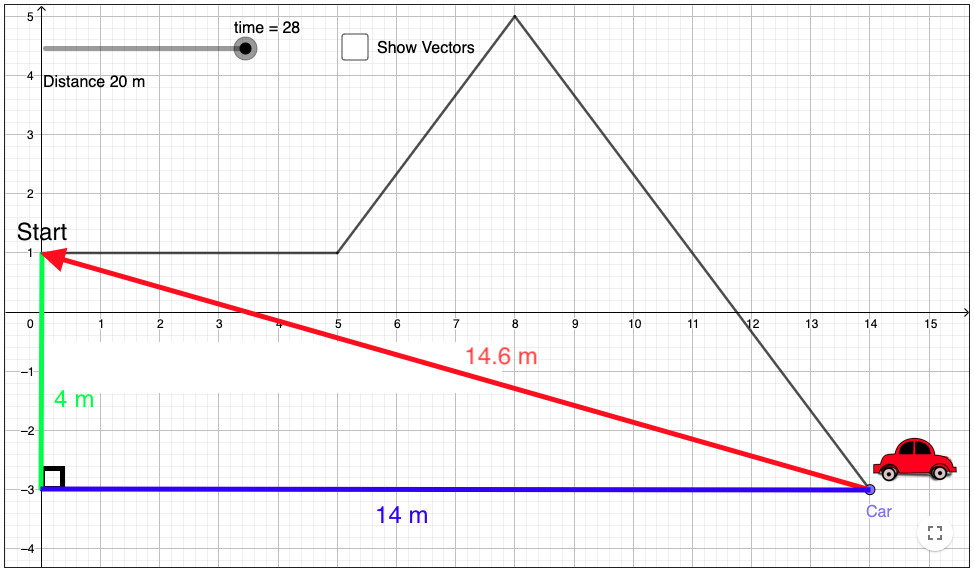# Average velocity vs speed - non-linear

Author:
Matt Dyck
Topic:
Vectors
The horizontal axis represents position in the east direction and the y axis represents the position in the north-south direction. Time is controlled using the slider. Moving the slider (time) causes the car to follow the path. Distance is how far the car travels and displacement is the vector from the car's starting position to it's current position.
Move the car along the first straight-away up to the first turn at time = 10 s. During that whole time, note that the distance travelled by the car iincreases continuously up to 5.0 m.

1. Continue to move the car through the rest of the path. Note that the distance travelled by the car increases the whole way. The second section, 10 s to 16 s, is also 5 m long. The third, 10 m long, section can be broken into two 5 m long sections (16s to 22s and 22s to 28s). Use the app to confirm those two distances.

2. Note the average speed calculation. It uses the total distance travelled and the total elapsed time. What do you notice about the speed during 10 s to 16 s compared to the first 10s?

3. Without moving the slider, calculate the average speed after 16 s, 22 s and 28s. Use the applet to confirm your answers afterward.

Return the time to 0 by using the slider or clicking the reset button at the top right.
Turn on the vectors if you have not already done so.

4. Moving the time from 0 s to 10 s, what do you notice about the distance and the magnitude of the displacement? What happens once the time increases past 10 s?

5. Move the time to 22 s. What do you notice about the displacement?

Notice the average velocity calculation. It uses the total displacement of the car and the total elapsed time.

6. What do you notice about the time used in the average distance and the time in the average velocity calculations?

7. Move the slider to 16 s. Use your vector skills to calculate the displacement magnitude and direction from the graph.

8. If the car completes his whole motion and then travels 14.6 m all the way back to the start in another 20 s as shown below, what would be the average speed?9. If the car completes his whole motion and then travels 14.6 m all the way back to the start in another 20 s as shown above, what would be the average velocity?